Home » A Practical Introduction to Prescriptive Analytics (with Case Study in R)

# A Practical Introduction to Prescriptive Analytics (with Case Study in R)

## Introduction

“What are the different branches of analytics?” Most of us, when we’re starting out on our analytics journey, are taught that there are two types – descriptive analytics and predictive analytics. There’s actually a third branch which is often overlooked – prescriptive analytics.

Prescriptive analytics is the most powerful branch among the three. Let me show you how with an example.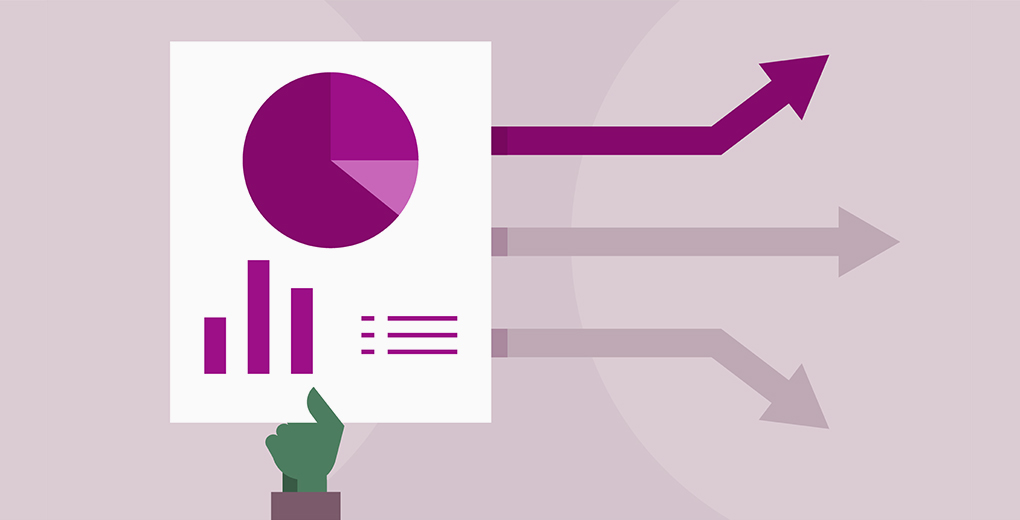Recently, a deadly cyclone hit Odisha, India, but thankfully most people had already been evacuated. The Odisha meteorological department had already predicted the arrival of the monstrous cyclone and made the life-saving decision to evacuate the potentially prone regions.

Contrast that with 1999, when more than 10,000 people died because of a similar cyclone. They were caught unaware since there was no prediction about the coming storm. So what changed?

The government of Odisha was a beneficiary of prescriptive analytics. They were able to utilize the services of the meteorological department’s accurate prediction of cyclones – their path, strength, and timing. They used this to make decisions about when and what needs to be done to prevent any loss of life.

So in this article, we will first understand what the term prescriptive analytics means. We will then solidify our learning by taking up a case study and implementing the branches of analytics -descriptive, predictive and prescriptive. Let’s go!

1. What is Prescriptive Analytics?
2. Setting up our Problem Statement
3. Hypothesis Generation
4. Laying Down our Model Building Approach
5. Data Visualization and Data Preparation – Descriptive Analytics
6. Prediction of Customer Behavior – Predictive Analytics
7. Recommendations to Improve Performance – Prescriptive Analytics

## What is Prescriptive Analytics?

We can broadly classify analytics into three distinct segments – Descriptive, Predictive and Prescriptive Analytics. Let’s take a look at each of these:

• Descriptive Analytics is the first part of any model building exercise. We analyze the historical data to identify patterns and trends of the dependent and independent variables. This stage also helps in hypothesis generation, variable transformation and any root cause analysis of specific behavioral patterns
• Predictive Analytics is the next stage of analytics. Here, we leverage the cleaned and/or transformed data and fit a model on that data to predict the future behavior of the dependent variable. Predictive analytics answers the question of what is likely to happen
• Prescriptive Analytics is the last stage where the predictions are used to prescribe (or recommend) the next set of things to be done. That’s where our Odisha Government example came from. They leveraged the predictions made by the meteorological department and took a series of measures, like relocating all people from low lying areas, arranging for food, shelter and medical help in advance, etc., to ensure the damage is limited

The below image does a nice job of illustrating the components under the prescriptive analytics umbrella:

## Setting up our Problem Statement

I’ve found the best way of learning a topic is by practicing it. So, let’s understand prescriptive analytics by taking up a case study and implementing each analytics segment we discussed above.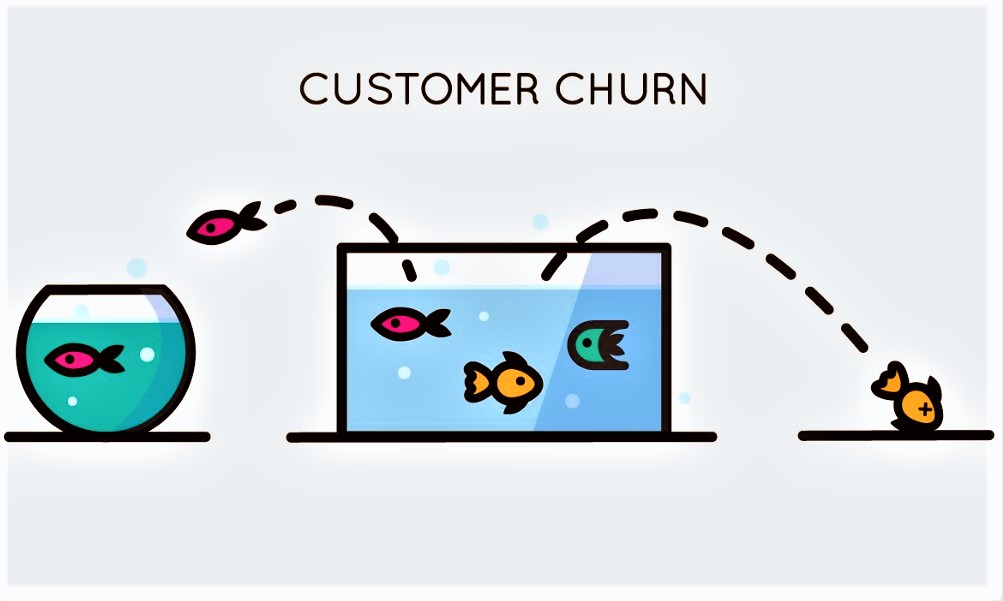The senior management in a telecom provider organization is worried about the rising customer attrition levels. Additionally, a recent independent survey has suggested that the industry as a whole will face increasing churn rates and decreasing ARPU (average revenue per unit).

The effort to retain customers so far has been very reactive. Only when the customer calls to close their account is when we take action. That’s not a great strategy, is it? The management team is keen to take more proactive measures on this front.

We as data scientists are tasked with analyzing their data, deriving insights, predicting the potential behavior of customers, and then recommending steps to improve performance.

## Getting the Dataset for our Problem

You can download the dataset from here. I have also provided the full code on my Github repositoryThere are three R files and you should use them in the below order:

## Hypothesis Generation

Generating a hypothesis is the key to unlocking any data science or analytics project. We should first list down what it is we are trying to achieve through our approach and then proceed from there.

Customer churn is being driven by the below factors (according the the independent industry survey):

• Cost and billing
• Network and service quality
• Data usage connectivity issues

We would like to test the same for our telecom provider. Typically, we encourage the company to come up with an exhaustive set of hypotheses so as not to leave out any variables or major points. However, we’ll narrow our focus down to one for the scope of this article:

Are the variables related to cost, billing, network, and service quality making a significant contribution towards a customer’s decision to stay with or leave the service provider?

## Laying Down our Model Building Approach

Now that we have the data set, the problem statement and the hypothesis to test, it’s time to get our hands dirty. Let’s tear into the data and see what insights can be drawn.

I have summarized my approach in the below illustration. Typically, any model building exercise will go through similar steps. Note that this is my approach – you can change things up and play around with the data on your end. For instance, we are removing variables with more than 30% missing values but you can take your own call on this.Here’s the code to find the variables with more than 30% missing values:

```mydata=read.csv("Telecom_Sampled.csv")
mydata\$churn=as.factor(mydata\$churn)
anyNA(mydata)
Percentage_missing=round(colSums(is.na(mydata[,colnames(mydata)[colSums(is.na(mydata))>0]]))/nrow(mydata)*100,2)
data.frame(MissingProportion=sort(Percentage_missing, decreasing = TRUE))

#Finding variable names with more than 30% missing values
Variables_with_High_NAs=colnames(mydata)[colSums(is.na(mydata))/nrow(mydata)>0.3]

#Removing the variables with more than 30% missing values from the original dataset
mydata=mydata[,!names(mydata)%in%Variables_with_High_NAs]

#13 variables removed
```

As you can see in the above illustration, we removed all variables with more than 30% missing values. Here’s the summary of our dataset: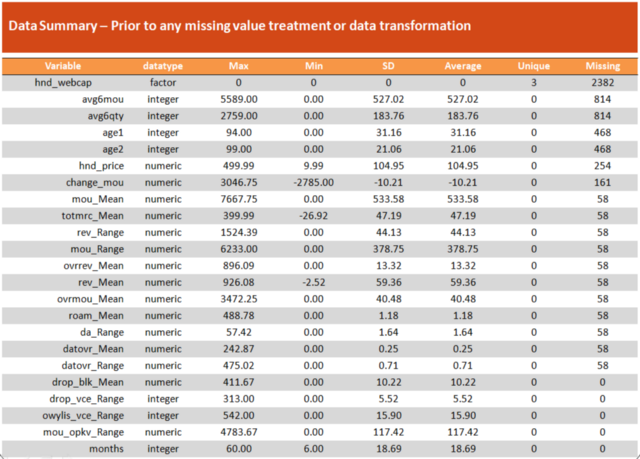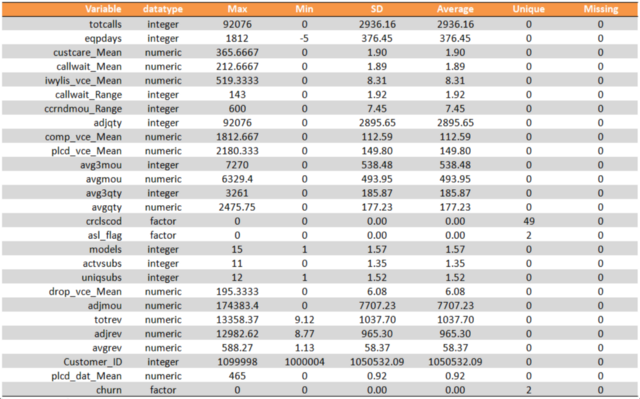We have reduced the number of variables from 82 to 69.

## Data Visualization and Data Preparation — Descriptive Analytics

Let’s do a univariate, bivariate and multivariate analysis of various independent variables along with the target variable. This should give us an idea of the effects of churn. I have shared a few visualizations below. You can find the entire exploratory analysis on the GitHub repository.

Let’s start by drawing up three plots (output is below the code block):

```#Univariate Analysis & Multivariate Analysis
a=ggplot(Telecom_Winsor, aes(x=mou_Mean, y=..density.., fill=1))
a+geom_histogram(stat = "bin", bins = 15)+geom_density(alpha=0.5)+
guides(fill=FALSE)+labs(y="Density", title="Density graph - MOU_Mean Vs Churn")+
theme_bw()+facet_grid(~churn)+theme(plot.title = element_text(size = 10, hjust = 0.5))

a=ggplot(Telecom_Winsor, aes(x=totmrc_Mean, y=..density.., fill=1))
a+geom_histogram(stat = "bin", bins = 15)+geom_density(alpha=0.5)+
guides(fill=FALSE)+labs(y="Density", title="Density graph - totmrc_Mean Vs Churn")+
theme_bw()+facet_grid(~churn)+theme(plot.title = element_text(size = 10, hjust = 0.5))

a=ggplot(Telecom_Winsor,aes(x=F_eqpdays), alpha=0.5)
a+geom_bar(stat = "count", aes(fill=models), position = "dodge")+
facet_grid(~churn)+labs(x="", fill="Models",y="Count", title="F_eqpdays Impact Churn?")+
theme(legend.position = c(0.8,0.8), plot.title = element_text(size = 10, hjust = 0.5),
legend.key.size = unit(0.5,"cm"), legend.title = element_text(size = 8),
legend.text = element_text(size = 8), axis.text.x = element_text( angle=45,size = 8, vjust = 1,
hjust = 1),
legend.direction = "horizontal")
```

First, we will analyze the mean minutes of usage, revenue range, mean total monthly recurring charge and the mean number of dropped or blocked calls against the target variable – churn: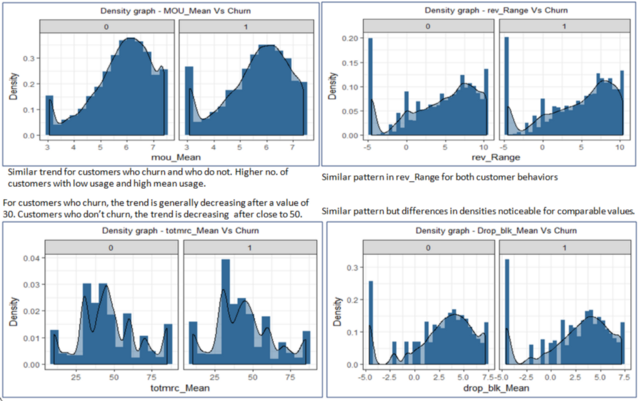Similarly, we shall analyze the mean number of dropped (failed) voice calls, the total number of calls over the life of the customer, the range of the number of outbound wireless to wireless voice calls and the mean number of call waiting against the churn variable: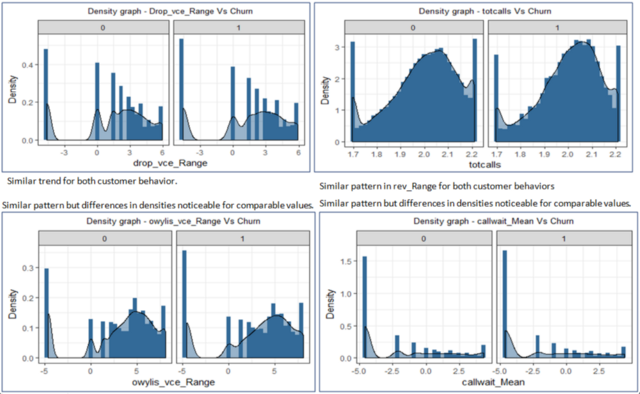Let’s change things up a bit. We’ll use the faceting functionality in the awesome ggplot2 package to plot the months of usage, credit class code, call drops and the number of days of current equipment against the churn variable: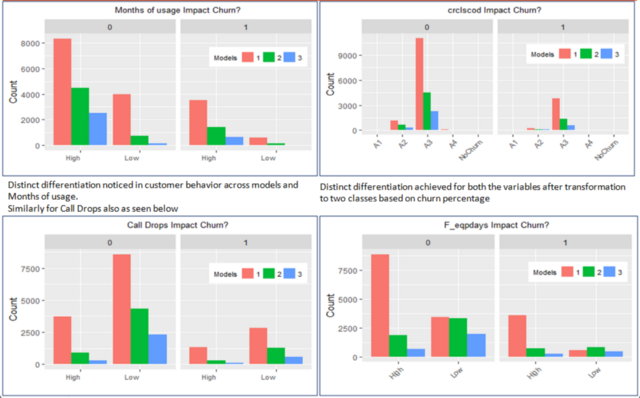We will analyze the numeric variable separately to see if there are any features that have high degrees of collinearity. This is because the presence of collinear variables always reduces the model’s performance since they introduce bias into the model.

We should handle the collinearity problem. Now, there are many ways of dealing with it, such as variable transformation and reduction using principal component analysis (PCA). I have removed the highly correlated variables:

```###Finding highly correlated variables
Numeric=Filter(is.numeric,mydata_Rev)
library(corrplot)
M=cor(na.omit(Numeric))
corrplot(M, method = "circle", type = "lower",
tl.srt = 45, tl.col = "black", tl.cex = 0.75)
```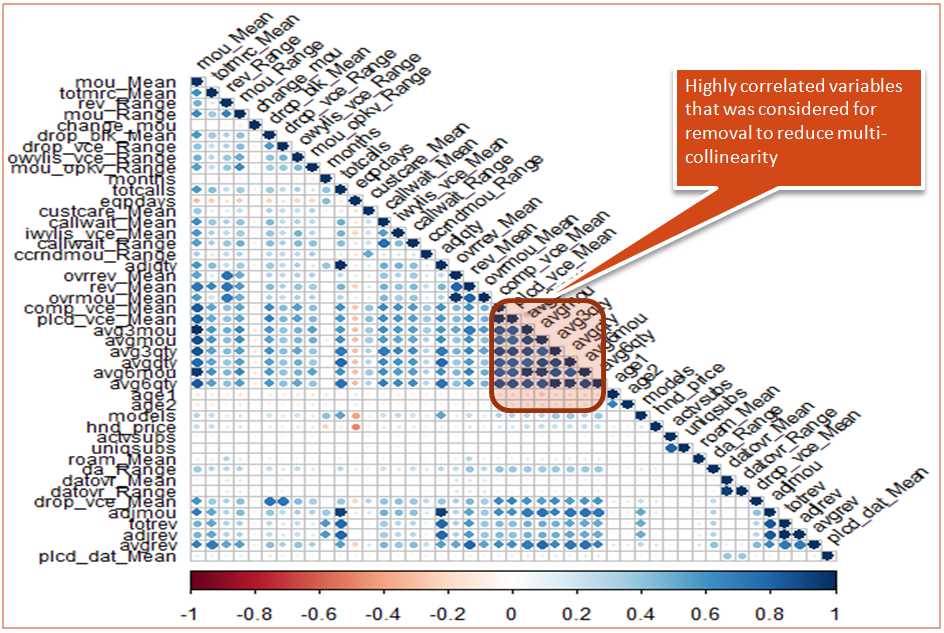## Prediction of Customer Behavior — Predictive Analytics

This is the part most of you will be familiar with – building models on the training data. We’ll build a number of models so we can compare their performance across the spectrum.

It is generally a good practice to train multiple models starting from simple linear models to complex non-parametric and non-linear ones. The performance of models varies depending on how the dependent and independent variables are related. If the relationship is linear, the simpler models give good results (plus they’re easier to interpret).

Alternatively, if the relationship is non-linear, complex models generally give better results. As the complexity of the model increases, the bias introduced by the model reduces and the variance increases. For our problem, we will build around ten models on the training set and validate them on unseen test data.

The models we’ll build are:

• Simple Models like Logistic Regression & Discriminant Analysis with different thresholds for classification
• Random Forest after balancing the dataset using Synthetic Minority Oversampling Technique (SMOTE)
• The ensemble of five individual models and predicting the output by averaging the individual output probabilities
• XGBoost algorithm

Here’s the code to the logistic regression model (you can try out the rest using the code provided in my GitHub repository):

```LGM1=glm(churn~., data = Telecom_Winsor, family = "binomial")
summary(LGM1)
#Remove hnd_wecap since it did not seem to be a significant and is introducing NAs in the model
names(Telecom_Winsor)
Telecom_Winsor_Lg=subset(Telecom_Winsor,select = -hnd_webcap)
Telecom_Winsor_Lg=droplevels(Telecom_Winsor_Lg)

#Data Splitting
library(caret)
set.seed(1234)

Index=createDataPartition(Telecom_Winsor_Lg\$churn,times = 1,p=0.75,list = FALSE)
Train=Telecom_Winsor_Lg[Index,]
Test=Telecom_Winsor_Lg[-Index,]

prop.table(table(Train\$churn))

LGM1=glm(churn~., data = Train, family = "binomial")
summary(LGM1)
step(LGM1, direction = "both")

library(car)

LGMF=glm(formula = churn ~ mou_Mean + totmrc_Mean + rev_Range + drop_blk_Mean +
drop_vce_Range + callwait_Mean + callwait_Range + ccrndmou_Range +
adjqty + rev_Mean + ovrmou_Mean + avgqty + age1 + age2 +
hnd_price + actvsubs + uniqsubs + datovr_Range + adjmou +
adjrev + plcd_dat_Mean + crclscod + asl_flag + mouR_Factor +
change_mF + F_months + F_eqpdays + F_iwylis_Vmean, family = "binomial",
data = Train)
car::vif(LGMF)
#adjmou, avgqty and adjqty have very high VIF but with Df considered GVIF is low enough. Hence no requirement for further collinesrity treatment

summary(LGMF)

Pred=predict(LGMF, Test, type = "response")
options(scipen = 9999)
L=data.frame(LogOfOdds=round(exp(coef(LGMF)),3))
L\$Variable=row.names(L)
row.names(L)=NULL
L=L[,c(2,1)]

#The variables which if undergo a change of 1 unit there is more than 50% probability of the customer decision changing from terminating service to staying with the servicer

L%>%arrange(desc(LogOfOdds))%>%filter(LogOfOdds>=1)%>%mutate(Probability=round(LogOfOdds/(1+LogOfOdds),3))

Pred.class=ifelse(Pred>0.24,1,0)
CM=confusionMatrix(as.factor(Pred.class),Test\$churn)
CM\$table
fourfoldplot(CM\$table)
Acc_Log24=CM\$overall[]
Sensitivity_Log24=CM\$byClass[]
Specificity_Log24=CM\$byClass[]
F1sq_Log24=CM\$byClass[]
library(ROCR)
Pred.Storage=prediction(Pred,Test\$churn)

AUC=performance(Pred.Storage,"auc")
[email protected][]
perf=performance(Pred.Storage,"tpr","fpr")

###########################

cut_offs=data.frame([email protected]alpha.values[], [email protected]s[], [email protected][])
cut_offs=cut_offs[order(cut_offs\$tpr, decreasing = TRUE),]
library(dplyr)
cut_offs%>%filter(fpr<=0.42,tpr>0.59)

#cutoff of 0.2356 seems to give the highest tpr and relatively low fpr

Pred.class235=ifelse(Pred>0.235,1,0)
CM=confusionMatrix(as.factor(Pred.class235),Test\$churn)
fourfoldplot(CM\$table)
Acc_Log23.5=CM\$overall[]
Sensitivity_Log23.5=CM\$byClass[]
Specificity_Log23.5=CM\$byClass[]

AUC=performance(Pred.Storage,"auc")
[email protected][]
F1sq_Log23.5=CM\$byClass[]
############
Pred.class=ifelse(Pred>0.25,1,0)
CM=confusionMatrix(as.factor(Pred.class),Test\$churn)
fourfoldplot(CM\$table)
Acc_Log25=CM\$overall[]
Sensitivity_Log25=CM\$byClass[]
Specificity_Log25=CM\$byClass[]
F1sq_Log25=CM\$byClass[]
AUC=performance(Pred.Storage,"auc")
[email protected][]
################################
Pred.class=ifelse(Pred>0.26,1,0)
CM=confusionMatrix(as.factor(Pred.class),Test\$churn)
fourfoldplot(CM\$table)
Acc_Log26=CM\$overall[]
Sensitivity_Log26=CM\$byClass[]
Specificity_Log26=CM\$byClass[]
F1sq_Log26=CM\$byClass[]
#Choice of cutoff at 24,25, 26 results in increasing accuracy and sensitivity but decreasing Specificity
AUC=performance(Pred.Storage,"auc")
[email protected][]```

Below is a comparison of the evaluation of our models: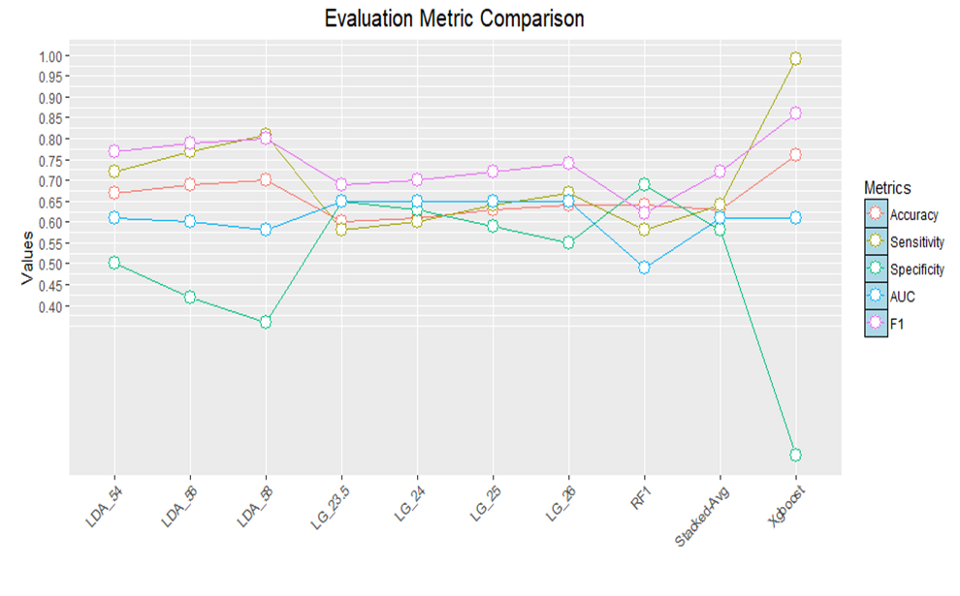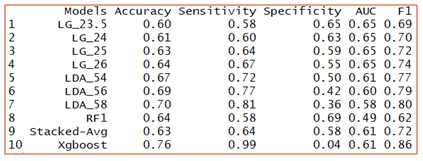Logistic regression seems to give the best result when compared with the other models. LG_26 is a logistic regression model with a threshold of 26%. Let me know if you improved on this score – I would love to hear your thoughts on how you approached this problem.

## Recommendations to improve performance — Prescriptive Analytics

And now comes the part we’ve been waiting for – prescriptive analytics! Let’s see what recommendations we can come up with to improve the performance of our model.

In the image below, we’ve listed the variables that have more than 50% probability of changing the decision of the customer for every 1 unit change in the respective independent variable. This insight was generated from the logistic regression model we saw above. That is essentially a relationship between the log of odds of the dependent variable with the independent variables.

So, if we calculate the exponential of coefficients of the dependent variable, we get the odds and from that, we get the probability (using formula Probability = Odds/(1+Odds)) of customer behavior changing for one unit change in the independent variable.

The below image will give you a better idea of what I’m talking about: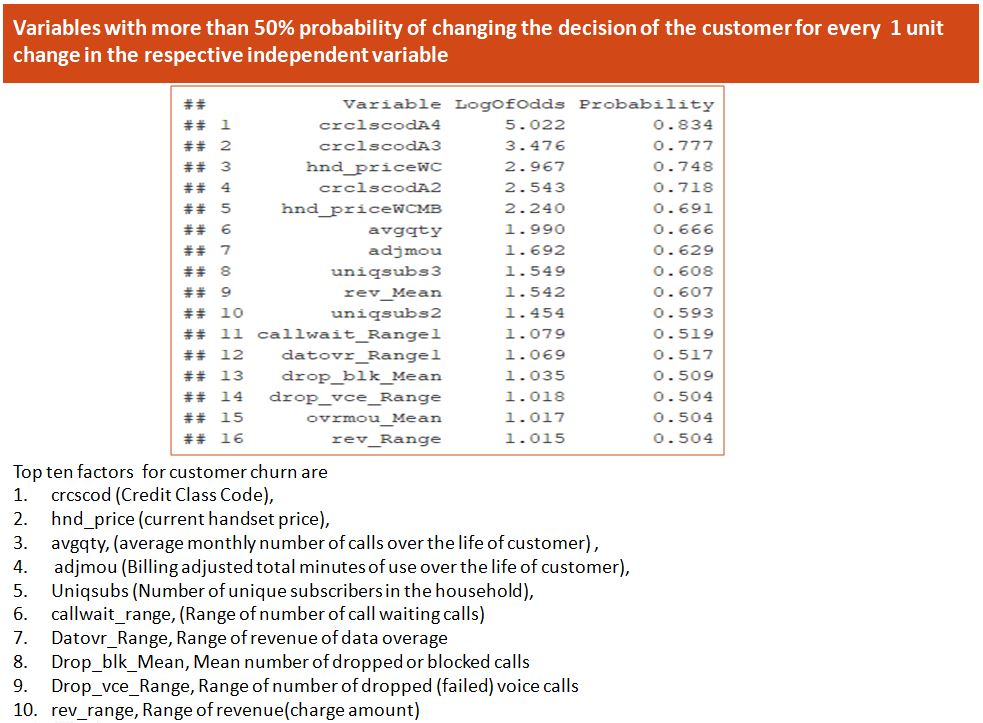Remember the hypothesis we generated using the independent survey earlier? This has also come out to be true. The below summary statistics from the logistic model proves that: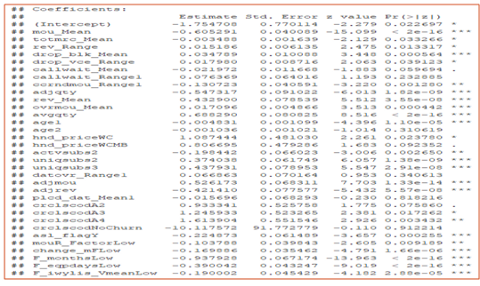Here’s a quick summary of what we can conclude from our analysis:

• Variables impacting cost and billing are highly significant
• Adjmou has one of the top 5 odds ratios
• The mean total monthly recurring charge (totmrc_Mean), Revenue (charge amount), Range (rev_Range), adjmou (Billing adjustments), etc. are found to be highly significant. This suggests that cost and billing impact customer behavior
• Similarly, network and service quality variables like drop_bkl_Mean (mean no. of dropped and blocked calls) is highly significant. Datovr_Range (Range of revenue of data overage) is not found to be significant but has an odds ratio of more than 1 indicating that 1 unit change in its value has more than a 50% chance of changing the customer behavior from one level to other. Perhaps we need to pay attention to it
• Additionally, the intercept is significant. This constitutes the effects of levels of categorical variables that were removed by the model

## Recommendations

Let’s pen down our recommendations based on what we’ve understood.

### Recommend rate plan migration as a proactive retention strategy

Mou_Mean (minutes of usage) is one of the most highly significant variables. Hence, it makes sense to work towards proactively working with customers to increase their MOU so that they are retained for a longer period.

Additionally, mouR_Factor is highly significant. This, remember, is a derived variable of mou_Range.

Changes in MOU are also highly significant. Change_mF is a derived variable of change_mou.

To complement the above, we also see that ovrmou_Mean is also a highly significant variable with an odds ratio of more than 1. The variable has a positive estimate of the coefficient indicating an increase in overage churn.

It would help if our company is able to work with the customers. Based on their usage, we can migrate them to optimal plan rates to avoid overage charges.

### Proactive retention strategy for customers

Identify customers who have the highest probability of churn and develop a proactive retention strategy for them. What if the budget is limited? Then the company can build a lift chart and optimize its retention efforts by reaching out to targeted customers:

Here, with 30% of the total customer pool, the model accurately provides 33% of total potential churn candidates: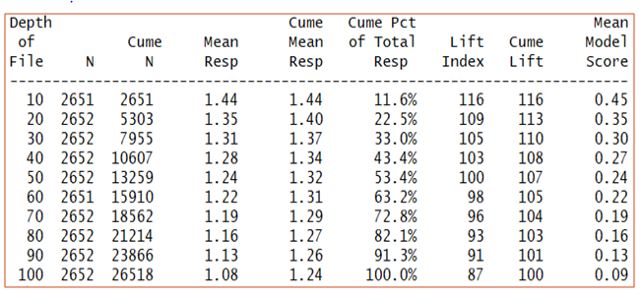The lift achieved will help us to reach out to churn candidates by targeting much fewer of the total customer pool with the company. Also notice how the first 30 deciles gives us the highest gain. This can give us around 33% of the customers who are likely to terminate the services.

In simple words, the company selects 30% of the entire customer database which covers 33% of the people who are likely to leave. This is much better than randomly calling customers which would have given perhaps a 15% hit rate from all potential churn candidates.

You can use the below code to test the model by identifying 20% of customers who need to be proactively worked with to prevent churn:

```gains(as.numeric(Telecom_Winsor\$churn),predict(LGMF,type="response",newdata=Telecom_Winsor[,-42])
,groups = 10)

Telecom_Winsor\$Cust_ID=mydata\$Customer_ID

Telecom_Winsor\$prob<-predict(LGMF,type="response",newdata=Telecom_Winsor[,-42])

quantile(Telecom_Winsor\$prob,prob=c(0.10,0.20,0.30,0.40,0.50,0.60,0.70,0.80,0.90,1))

targeted=Telecom_Winsor%>%filter(prob>0.3224491 & prob<=0.8470540)%>%dplyr::select(Cust_ID)
```

They are the customers whose probability of churn is greater than 32.24% and less than 84.7%. The ModelBuilding.r code will help you with the logical flow of the above code block.

## End Notes

Prescriptive analytics is a truly awesome thing if companies are able to utilize it properly. It’s still under the radar as far as the three branches of analytics are concerned.

But as we keep moving up in the hierarchy of analytics, prescriptive analytics is the most favored area as it can help organizations to plan and prepare as they can foresee the future with a fair degree of confidence.

Prescriptive analytics seeks to determine the best solution or outcome among various choices. Just keep in mind that we cannot separate the three branches of analytics. We need to do descriptive and predictive before jumping into prescriptive.

Pranov Mishra

Pranov is a Data Science enthusiast with about 11 years of professional experience in the Financial Services industry. Pranov is working as a Vice President in a Multinational Bank and has exposure to Strategic Planning, Intelligent Automation, Data Science, Risk & Controls, Predictive Data Modelling, and People Management. He also mentors analytics (PGPBABI) students enrolled with Great Learning and Great Lakes.

You can also read this article on our Mobile APP•Vinay Gupta says:

That’s fantastic!
Loved the simplicity of explanation. Kudos Parnov.

•Pranov Shobhan Mishra says:

Thanks, Vinay. Glad that you liked it.

•bharath says:

Great Post !!!

•Ritesh says:

Great article. Where can i find the description about the column headers??

•Ritesh says:

where can we find the expanded form of all the variables?

•Pabitra says:

Very informative and comprehensive article. Thanks

•Kartik says:

Nice one

•Krishna Mohan says:

Excellent write up Pranov. In addition to making your point about Prescriptive Analytics, you have also outlined how to systematically perform Descriptive and Predictive Analytics as well. A must read for any Analytics student.

•Pranov Mishra says:

Thanks Krishna Mohan. ENcouraging

•Basavaraj Mayee says:

Nice one Pranov. You started with nice example.

•Sneha Gorle says:

Nice Post Pranov